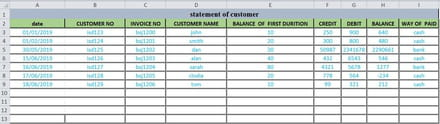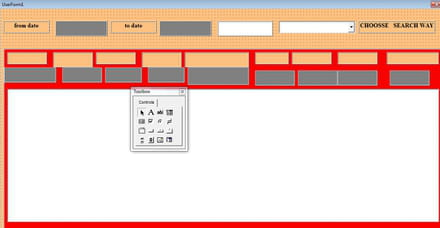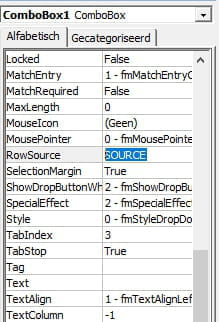# Fixing complicated error populate data in listbox based on combobox and textbox

Solved/Closed
abdelfatah_0230 Posts 73 Registration date Thursday July 18, 2019 Status Member Last seen July 23, 2022 - Updated on Jul 19, 2020 at 06:13 AM
abdelfatah_0230 Posts 73 Registration date Thursday July 18, 2019 Status Member Last seen July 23, 2022 - Aug 7, 2020 at 07:07 AM
Hello,
actually theses codes works very well in specific workbook i copy theses and adjusted what i need ranges names ..etc but it show me error permission denied in this line
.Column = sRng.Value my programs works this choose from combobox based on specific column then i write in textbox1 and supposing show data in listbox
indeed i follow the lines code line by line but i don't reach any result so i hope any body help to check the codes and correct me the mistake
`Private Const ContColmn As Integer = 9Private Const DateFormt As String = "yyyy/mm/dd"Private sRng As RangePrivate sColmnPrivate Sub ComboBox1_Change()Dim vbol As Booleanvbol = CBool(Me.ComboBox1.ListIndex + 1 = 3)Me.TextBox1.Visible = Not vbolEnd Sub`

`Private Sub TextBox1_Change()Dim MyValueDim MyAr() As StringDim ib As BooleanDim R As Integer, i As Integer, ii As IntegerDim MyColmnFind As Integer, Lastrow As IntegerDim dt1 As Date, dt2 As Date'-------------------------MyColmnFind = Me.ComboBox1.ListIndex + 1If MyColmnFind = 0 Then Exit SubIf MyColmnFind = 3 Then Me.TextBox1 = ""'-------------------------Me.ListBox1.Clear'-------------------------With sRng.Worksheet    Lastrow = .Range("A65536").End(xlUp).Row    If IsDate(Me.textbox1) Then dt1 = DateValue(Me.textbox1) Else dt1 = WorksheetFunction.Min(.Range("a3").Resize(Lastrow)): Me.textbox1= Format(dt1, DateFormt)    If IsDate(Me.textbox2) Then dt2 = DateValue(Me.textbox2) Else dt2 = WorksheetFunction.Max(.Range("a3").Resize(Lastrow)): Me.textbox2= Format(dt2, DateFormt)End With'-------------------------sColmn = ""With sRng    For R = 2 To Lastrow        Select Case .Cells(R, 1).Value2: Case dt1 To dt2            ib = InStr(1, .Cells(R, MyColmnFind), Me.TextBox1, vbTextCompare) = 1            If ib Then                sColmn = sColmn & R & " "                ii = ii + 1                ReDim Preserve MyAr(1 To ContColmn, 1 To ii)                For i = 1 To ContColmn                    If IsDate(.Cells(R, i)) Then MyValue = Format(.Cells(R, i).Value2, DateFormt) _                    Else MyValue = .Cells(R, i).Value2                    MyAr(i, ii) = MyValue                Next            End If        End Select    NextEnd WithIf ii Then Me.ListBox1.Column = MyAr: Me.ListBox1.ListIndex = 0end sub`

`Private Sub UserForm_Activate()Dim wColmn'-----------------------------Set sRng = sheet1.Range("A2").Resize(1, ContColmn)'-----------------------------For i = 1 To ContColmn    With Me.Controls("Lab" & i)        .Caption = sRng(i)        wColmn = wColmn & .Width & " "    End WithNext'-----------------------------wColmn = Join(Split(Trim(wColmn)), ",")'-----------------------------With Me.ListBox1    .ColumnCount = ContColmn    .ColumnWidths = wColmnEnd With'-----------------------------With Me.ComboBox1    .Column = sRng.Value    .ListIndex = 0    .Style = 2End With'-----------------------------'Me.ButtonSaveFil.Enabled = False'Me.ButtonSelect.Enabled = FalseEnd Sub`

datat sheetuserform## 5 replies

TrowaD Posts 2900 Registration date Sunday September 12, 2010 Status Moderator Last seen September 12, 2022 523
Aug 4, 2020 at 12:16 PM
Hi Abdel,

You are right. The problem is data types. You have 3: Text, number and date. We need to check them individualy. Doing that creates another problem; comparing a, for example, number as date creates an error.

A short story shorter; a lot of error handling added.

Here is your code:
```Private Sub CommandButton2_Click()
Dim MyValue
Dim MyAr() As String
Dim ib As Boolean
Dim R As Integer, i As Integer, ii As Integer
Dim MyColmnFind As Integer, Lastrow As Integer
Dim dt1 As Date, dt2 As Date
'-------------------------
MyColmnFind = Me.ComboBox1.ListIndex + 1
If MyColmnFind = 0 Then Exit Sub
If MyColmnFind = 3 Then Me.TextBox10 = ""
'-------------------------
Me.ListBox1.Clear
'-------------------------
With sRng.Worksheet
Lastrow = .Range("A65536").End(xlUp).Row
If IsDate(Me.DTPicker1) Then dt1 = DateValue(Me.DTPicker1) Else dt1 = WorksheetFunction.Min(.Range("a3").Resize(Lastrow)): Me.DTPicker1 = Format(dt1, DateFormt)
If IsDate(Me.DTPicker2) Then dt2 = DateValue(Me.DTPicker2) Else dt2 = WorksheetFunction.Max(.Range("a3").Resize(Lastrow)): Me.DTPicker2 = Format(dt2, DateFormt)
End With

'-------------------------
sColmn = ""
With sRng
For R = 2 To Lastrow
On Error GoTo NextCheck ' When the line below creates a type mismatch error because the textbox value isn't a date then got to line NextCheck
If .Cells(R, MyColmnFind).Value = DateValue(TextBox1.Value) Then GoTo addArr ' When there isn't an error and the data matches, then continue to add data row to MyAr
NextCheck:
On Error GoTo -1 ' Resets error
On Error GoTo NextCheck2 ' When the line below creates a type mismatch error because the textbox value isn't a number then got to line NextCheck2
If .Cells(R, MyColmnFind).Value = CInt(TextBox1.Value) Then GoTo addArr
NextCheck2:
On Error GoTo -1
If .Cells(R, MyColmnFind).Value = TextBox1.Value Then
Select Case .Cells(R, 1).Value: Case dt1 To dt2
ib = InStr(1, .Cells(R, MyColmnFind), Me.TextBox10, vbTextCompare) = 1
If ib Then
sColmn = sColmn & R & " "
ii = ii + 1
ReDim Preserve MyAr(1 To ContColmn, 1 To ii)
For i = 1 To ContColmn
If IsDate(.Cells(R, i)) Then MyValue = Format(.Cells(R, i).Value, DateFormt) _
Else MyValue = .Cells(R, i).Value
MyAr(i, ii) = MyValue
Next
End If
End Select
End If
Next
End With
If ii Then Me.ListBox1.Column = MyAr: Me.ListBox1.ListIndex = 0

End Sub```

Hopefully that covers all the bases :)

Best regards,
Trowa
abdelfatah_0230 Posts 73 Registration date Thursday July 18, 2019 Status Member Last seen July 23, 2022
Aug 7, 2020 at 07:07 AM
yes, Trowa now your updating works greatly , good job buddy

Best regards,
abdelfattah
grorge441 Posts 24 Registration date Tuesday July 7, 2020 Status Member Last seen April 24, 2021 15
Jul 22, 2020 at 03:18 AM
abdelfatah_0230 Posts 73 Registration date Thursday July 18, 2019 Status Member Last seen July 23, 2022
Updated on Jul 22, 2020 at 04:20 AM
thanks grorge for your trying to help me actually , your suggestion is the same thing somebody advised me but i think the problem is not the system in windows i thing the problem is in the code because i run this original code in the same pc system win7 works normally when i changed the ranges and the names sheets, tools in userform it gives me error

TrowaD Posts 2900 Registration date Sunday September 12, 2010 Status Moderator Last seen September 12, 2022 523
Jul 23, 2020 at 11:22 AM
Hi Abdel,

Could you post your workbook? I will see what I can do.

Best regards,
Trowa
abdelfatah_0230 Posts 73 Registration date Thursday July 18, 2019 Status Member Last seen July 23, 2022
Jul 23, 2020 at 03:09 PM
TrowaD Posts 2900 Registration date Sunday September 12, 2010 Status Moderator Last seen September 12, 2022 523
Jul 28, 2020 at 11:48 AM
Hi Abdel,

Your ComboBox1 has a RowSource value. Remove the value SOURCE as seen in the screengrab below:Best regards,
Trowa
abdelfatah_0230 Posts 73 Registration date Thursday July 18, 2019 Status Member Last seen July 23, 2022
Updated on Jul 28, 2020 at 02:26 PM
yes , it works but i need your help about another problem when i choose from combobox customer name and write name it shows me all of data it supposes showing only data of specific name written in textbox 1 also the same problem if i choose invoice no it shows all of data not data of specific invoice

Didn't find the answer you are looking for?

TrowaD Posts 2900 Registration date Sunday September 12, 2010 Status Moderator Last seen September 12, 2022 523
Jul 30, 2020 at 11:40 AM
Hi Abdel,

For that request I added an IF statement to the code behind the search button. The green text shows what I changed/added.

Here is the code:
```Private Sub CommandButton2_Click() ' ***** Changed 3 to 2 since there is no CommandButton3
Dim MyValue
Dim MyAr() As String
Dim ib As Boolean
Dim R As Integer, i As Integer, ii As Integer
Dim MyColmnFind As Integer, Lastrow As Integer
Dim dt1 As Date, dt2 As Date
'-------------------------
MyColmnFind = Me.ComboBox1.ListIndex + 1
If MyColmnFind = 0 Then Exit Sub
If MyColmnFind = 3 Then Me.TextBox10 = ""
'-------------------------
Me.ListBox1.Clear
'-------------------------
With sRng.Worksheet
Lastrow = .Range("A65536").End(xlUp).Row
If IsDate(Me.DTPicker1) Then dt1 = DateValue(Me.DTPicker1) Else dt1 = WorksheetFunction.Min(.Range("a3").Resize(Lastrow)): Me.DTPicker1 = Format(dt1, DateFormt)
If IsDate(Me.DTPicker2) Then dt2 = DateValue(Me.DTPicker2) Else dt2 = WorksheetFunction.Max(.Range("a3").Resize(Lastrow)): Me.DTPicker2 = Format(dt2, DateFormt)
End With

'-------------------------
sColmn = ""
With sRng
For R = 2 To Lastrow
If .Cells(R, MyColmnFind).Value2 = TextBox1.Value Then ' ***** Added an IF statement to check if data row needs to be added to MyAr
Select Case .Cells(R, 1).Value2: Case dt1 To dt2
ib = InStr(1, .Cells(R, MyColmnFind), Me.TextBox10, vbTextCompare) = 1
If ib Then
sColmn = sColmn & R & " "
ii = ii + 1
ReDim Preserve MyAr(1 To ContColmn, 1 To ii)
For i = 1 To ContColmn
If IsDate(.Cells(R, i)) Then MyValue = Format(.Cells(R, i).Value2, DateFormt) _
Else MyValue = .Cells(R, i).Value2
MyAr(i, ii) = MyValue
Next
End If
End Select
End If ' ***** Added line
Next
End With
If ii Then Me.ListBox1.Column = MyAr: Me.ListBox1.ListIndex = 0

End Sub```

Best regards,
Trowa
abdelfatah_0230 Posts 73 Registration date Thursday July 18, 2019 Status Member Last seen July 23, 2022
Jul 30, 2020 at 02:34 PM
your code works only when i select customer name but the rest of choices in combobox doesn't show any thing like invoice no or customer no ..etc i hope you can fix it

best regards

abdelfattah